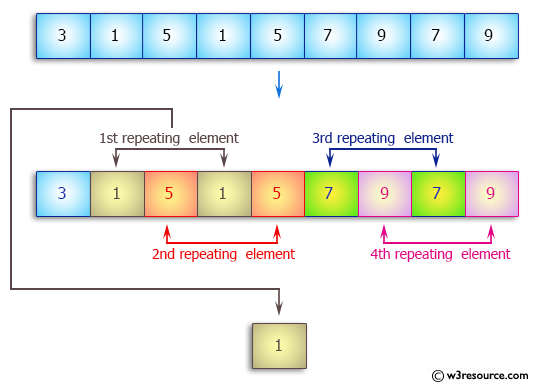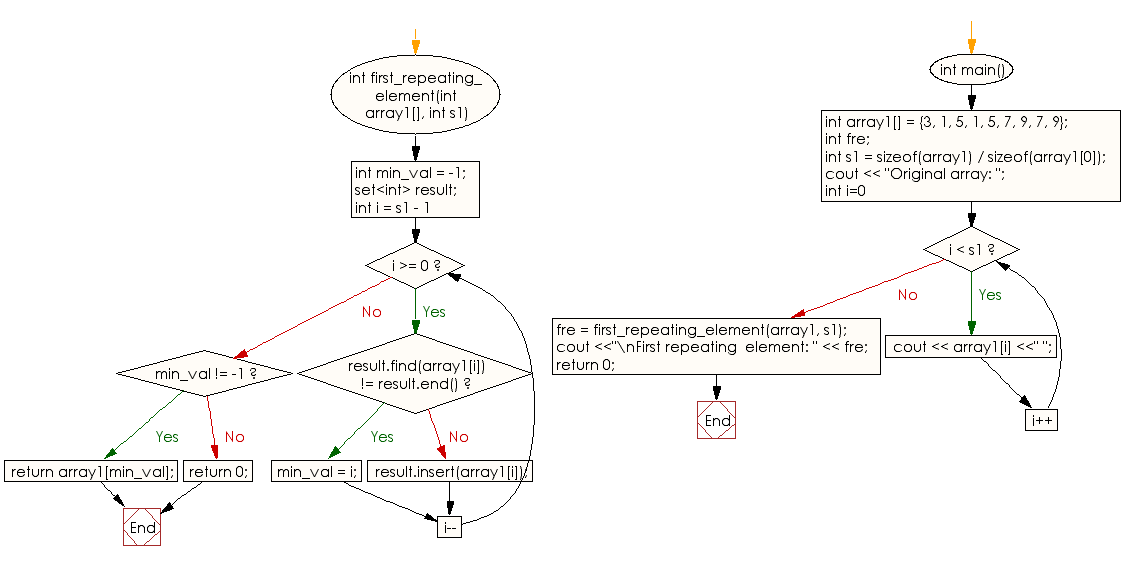﻿ C++ : Find the first repeating value in an array of integers# C++ Exercises: Find the first repeating element in an array of integers

## C++ Array: Exercise-24 with Solution

Write a C++ program to find the first repeating element in an array of integers.

Pictorial Presentation:Sample Solution:

C++ Code :

``````#include<iostream>
using namespace std;
int first_repeating_element(int array1[], int s1)
{
int min_val = -1;

set<int> result;

for (int i = s1 - 1; i >= 0; i--)
{
if (result.find(array1[i]) != result.end())
min_val = i;

else
result.insert(array1[i]);
}

if (min_val != -1)
return array1[min_val];
else
return 0;
}

int main()
{
int array1[] = {3, 1, 5, 1, 5, 7, 9, 7, 9};
int fre;

int s1 = sizeof(array1) / sizeof(array1);

cout << "Original array: ";

for (int i=0; i < s1; i++)
cout << array1[i] <<" ";

fre = first_repeating_element(array1, s1);
cout <<"\nFirst repeating  element: " << fre;
return 0;
}
``````

Sample Output:

```Original array: 3 1 5 1 5 7 9 7 9
First repeating  element: 1
```

Flowchart:C++ Code Editor:

What is the difficulty level of this exercise?

Test your Programming skills with w3resource's quiz.

﻿